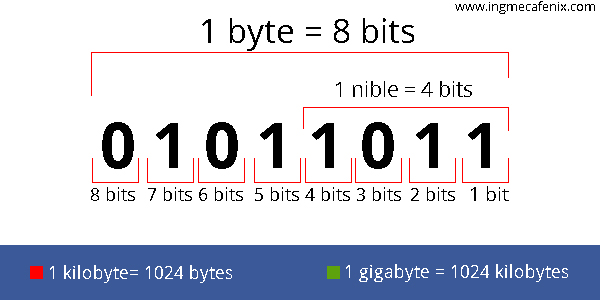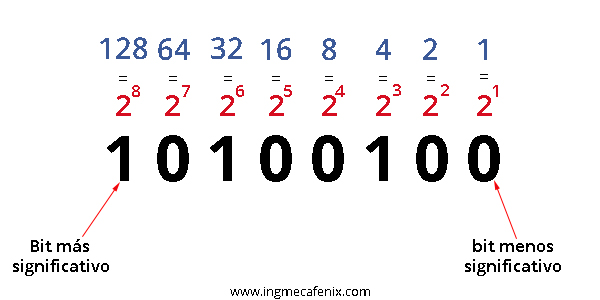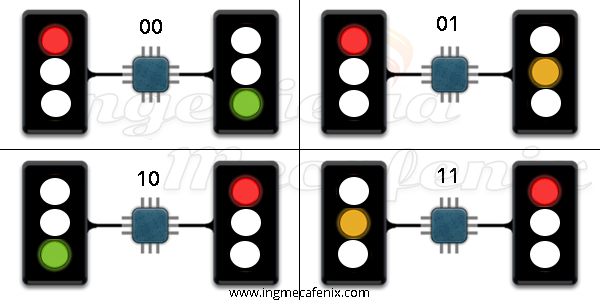#The binary code is basically a new number system with two unique variables such as number 1 (for active state) and 0 (for deactivated), despite the fact that there are only two symbols we can create an infinity of combinations since we can reuse them as many times as necessary.

The binary system has a way of classifying the data it represents, such as the bit, the byte, the kilobyte, the megabyte, the gigabyte, etc. In reference in the decimal system we classify them as units, tens, hundreds, etc.## What does binary code represent?

The binary system becomes code when we start to make combinations, these can represent anything, be it data or numbers.

### Representation of numbers

To represent the decimal numbers that we are used to using, we have to know two basic rules.

• First rule: The first bit equals 2 ^ 0, the second bit equals 2 ^ 1, the third bit equals 2 ^ 2, and so on. In other words, the first bit equals 1, the second bit equals twice the previous one, that is, it equals 2, for the third the double of 2 is 4.
• Second rule: Numbers are read from the most significant bit (msb) to the least significant bit (lsb), in other words from right to left.Examples

This time we will represent the numbers 1, 3, 13 and 28 in binary numbers.### Data representation

The data representation is used in such a way that each of the combinations is equivalent to a specific data.

For example:

To represent 6 different colors with binary code we need to use 6 different combinations. To know how many possible combinations a bit has, we only have to solve 2 ^ number of bits, example possible combinations in 2 bits 2 ^ 2 = 2 * 2 = 4 the combinations are not necessary, 2 ^ 3 = 2 * 2 * 2 = 8 possible combinations, with three bits we can use up to 8 different possible combinations.### Binary representation in electronics

The representation of binary code in electronics is very similar to that of data since digital electronic circuits only work with 2 states: 1 when there is voltage and 0 when there is not.

For example: if we wanted to represent a junction with two semaphores we would need 4 different combinations which is equivalent to 2 bits. To represent the green of a traffic light and the red of the other we only need 1 state since this is executed in parallel, the same thing happens with the yellow of a traffic light and red.

### Combinations

• «00»: traffic light 1 is programmed in red and 2 in green.
• «01»: traffic light 1 remains red and 2 changes to yellow.
• «10»: traffic light 1 turns green and 2 turns red.
• «11»: traffic light 1 changes to yellow and 2 continues to red.